# Construct surface mesh¶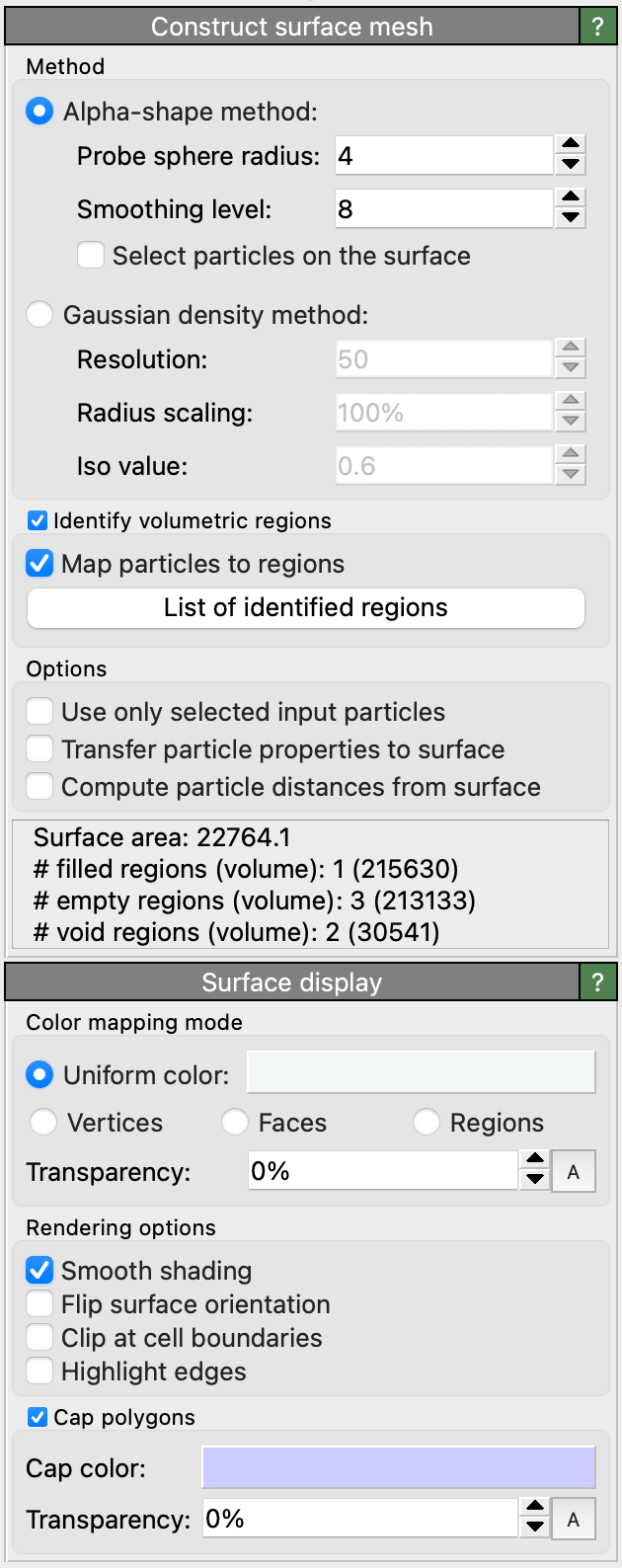This modifier constructs a surface representation of the three-dimensional shape formed by a set of particles. It can thus generate a geometric description of the outer and inner boundaries of an atomistic solid in terms of a triangulated surface mesh. Besides visualization purposes the geometric description of the surface is also useful for quantitative measurements of the surface area and the solid volume and porosity of an atomistic structure.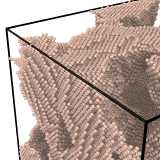InputOutput

## Introduction¶

The modifier offers two alternative surface construction methods: The alpha-shape method and the Gaussian density method, which will be described in more detail in later sections. Both approaches have specific advantages and limitations. The alpha-shape method assumes that all particles are point-like, i.e., the atomic radii are ignored. Instead the concept of a virtual probe sphere of finite size is used to define the accessible spatial volume (i.e. the empty, exterior region), the inaccessible volume (the solid, interior region) and the separating surface between the two. This approach provides the capability to quantify filled and empty volumes or to analyze the porosity of a structure, for example. Furthermore, it exactly specifies which particles are located right at the surface, making it a well-defined criterion for selecting surface atoms.

The second method offered by the modifier is based on a smeared-out representation of the finite-sized particle spheres in terms of overlapping Gaussian distribution functions centered at each particle. The resulting density field, which is computed on a discrete grid, has local maximums at each particle site and decays to zero far away from any particles. Next, the surface boundary is constructed as an isosurface of the Gaussian density field, with the threshold chosen such that the resulting isosurface roughly matches the finite diameters of the spherical input particles. This approach thus provides the advantage of accounting for the finite extent of the particles themselves, which is important for small molecules that do not form a bulk structure and in which a majority of atoms is at the surface.

## General options¶

The modifier provides the option to operate only on the subset of currently selected input particles. This allows you to exclude certain parts of the system from the surface construction if needed. For example, to ignore water molecules surrounding the structure of interest.

The total surface area of the resulting manifold is displayed by the modifier in the status area and output as a global attribute named ConstructSurfaceMesh.surface_area. Note that the surface area is given in squared units of length as used by the original simulation dataset.

OVITO has the capability to associate local information with the surface mesh, which gets inherited from nearby particles during the construction process. If the option Transfer particle properties to surface is turned on, existing attributes of the input particles located at the surface, for example their Color property, will be copied over to the vertices of the constructed surface mesh. The per-particle values will be available as mesh vertex properties, and you can subsequently use tools like the Color coding modifier to further manipulate the surface mesh, independently of the underlying particles.

Note that, in case of the Gaussian density method, only continuous particle properties of data type Float will be transferred to the surface. Discrete integer properties will be ignored, because the algorithm has to blend the property values of several particles to compute the value at each output mesh vertex. In case of the alpha-shape method, all types of properties can be transferred, because there is a one-to-one mapping between particles and mesh vertices.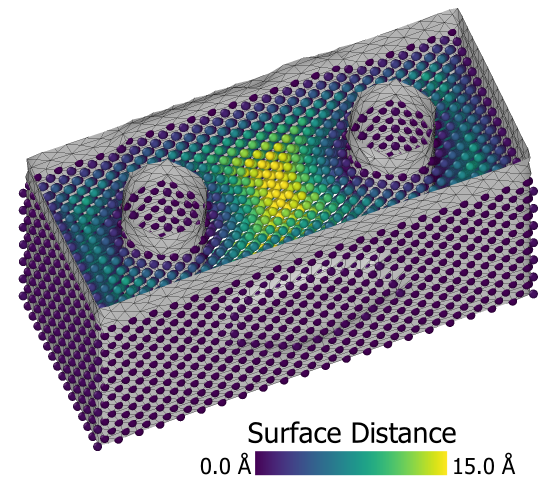Upon request, the modifier can compute the distance of each particle to the closest point on the constructed surface. The computed distances are stored as a new particle property named Surface Distance, which may subsequently be used to select particles within a certain range from the surface, e.g. using the Expression selection modifier. Note, however, that the distance calculation is a very expensive operation, which can take a long time for systems with many particles or a complex surface. If you just want to select particles located exactly on the surface, then the option Select particles on the surface should be used instead. It is only available for the alpha-shape method and lets the modifier directly select particles that are part of the surface mesh, i.e. which are touched by the virtual probe sphere.

## Periodic systems and cap polygons¶OVITO correctly handles systems with periodic boundary conditions (including mixed open and periodic conditions). The simulation box here constitutes a periodic domain where the surface manifold is embedded in. The constructed surface may wrap around at the box boundaries and even reconnect to itself to form an infinite manifold. Furthermore, there are two particular situations where the surface will turn out degenerate (i.e. a mesh with no faces): (i) A simulation box containing no particles or without particles forming any solid region, and (ii) a periodic simulation box densely and completely filled with particles. In case (i) there will be single space-filling empty region and in case (ii) a single space-filling solid region. OVITO differentiates between the two degenerate cases when it comes to visualization of cap polygons.

For visualization a surface cut by a periodic simulation box boundary is wrapped around and reenters on the opposite side of the box. For the sake of clarity, the surface mesh visual element of OVITO provides the option to render so-called cap polygons to close the holes in the surface that occur due to these cuts at the box boundaries. Cap polygons make it easier for an observer to discern solid regions from empty regions.

## How the alpha-shape algorithm works¶

The procedure for surface reconstruction from a set of point-like particles or atoms is described in

which is also the reference you should cite when publishing quantitative results obtained with this analysis tool. The method makes use of a Delaunay tessellation constructed on the basis of the input particle coordinates. It tessellates space into tetrahedral simplices, which are subsequently classified as either belonging to a filled spatial region or an empty spatial region. Finally, the surface manifold is constructed as the separating boundary between the empty and the filled regions of space.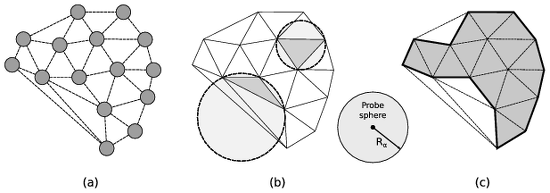(a) The Delaunay tessellation calculated from the input point set. (b) The circumscribed spheres of two exemplary tessellation elements are highlighted. One element’s circumsphere is larger than the probe sphere while the other one is smaller. Consequently, the elements are classified as open and solid, respectively. (c) The union of all solid Delaunay elements defines the geometric shape of the atomistic solid (bold line).

The concept of a probe sphere with a prescribed radius (alpha parameter) is employed to assign each tetrahedral Delaunay element to either the empty or the filled region. Generally, the empty region is defined as the union of all locations in space that are accessible to the virtual probe sphere without touching any of the input particle centers. Note that this includes any cavities inside the solid too as long as they can accommodate the virtual sphere without it overlapping with any particles. The radius of the probe sphere is a length scale parameter that determines how much detail and small features (e.g. pores) of the geometric shape will be captured by the method.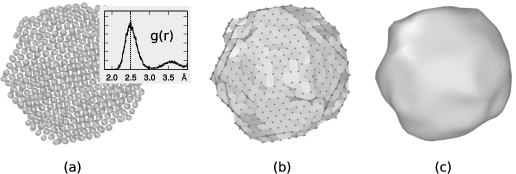(a) Atomistic model of a carbon nanoparticle with an inset showing the corresponding pair distribution function. The position of the first peak is used as probe sphere radius ($$R_{\alpha}=2.5 \mathrm{\AA}$$) for the alpha-shape construction. (b) The resulting triangulated surface mesh. (c) Final surface model after six iterations of the smoothing algorithm were applied.

The alpha-shape method, originally introduced by Edelsbrunner and Mücke [ACM Trans. Graph. 13:43–72, 1994], compares the circumscribed spheres of the tetrahedral Delaunay elements (figure b) to the probe sphere to efficiently decide which regions of space can accommodate the probe sphere without any overlap and which thus become part of the empty spatial region. The remaining tetrahedra form the filled (solid) region. Finally, the closed surface mesh can be constructed, which consists of all triangular faces of the three-dimensional Delaunay tessellation that separate solid from open tetrahedra (figure (c)).

The resulting surface mesh still reflects the atomic steps that are typically present on the surfaces of an atomistic object. The contribution of surface steps may lead to an overestimation of the macroscopic surface area. Therefore one can subsequently apply a fairing procedure [Taubin, SIGGRAPH 95 Conf. Proc., pp. 351-358, 1995] to the surface mesh to smoothen out the steps.

It should be emphasized that the results of the described surface reconstruction method will generally depend on the selected probe sphere radius parameter $$R_{\alpha}$$. A rule of thumb for choosing $$R_{\alpha}$$ is to use the nearest neighbor atom separation distance in the material at hand as demonstrated in figure (a) on the right. OVITO allows you determine the first peak of the radial pair distribution function $$g(r)$$ with the Coordination analysis modifier if needed. Generally, you should report the value of $$R_{\alpha}$$ in your publications when measuring surface area, solid volumes, or porosities.

## Parameters¶

This parameter controls the radius of the probe sphere used in the surface construction algorithm ($$R_{\alpha}$$), which determines the level of surface details captured. A larger sphere radius leads to a surface with less details, which reflects only coarser features of the surface topology. A small sphere radius, on the other hand, will resolve finer surface features and small voids inside a solid. However, the sphere radius should not be smaller than half of the typical interatomic distance. Otherwise artificial holes will appear in the constructed surface.

A rule of thumb is to choose this parameter equal to the nearest neighbor atom separation in the material at hand. You can use the Coordination analysis modifier to determine the nearest neighbor separation, which is the position of the first peak in the radial pair distribution function.

Smoothing level

After the triangulated surface mesh has been constructed, it is further refined by applying a smoothing and fairing algorithm to remove atomically sharp surface steps. This parameter controls how many iterations of the smoothing algorithm are performed.

Identify volumetric regions pro

This option lets the modifier identify the individual spatial regions enclosed by the surface manifold (both empty and filled regions). Each region’s volume and surface area are computed and output as a property list attached to the surface mesh. See the next section for details.

## Identification of volumetric regions pro¶A periodic 2d structure consisting of two empty regions (pores) and two filled regions.

The modifier provides the option (incurring some extra computational cost) to identify the spatial regions bounded by the surface manifold and to compute the respective volume and surface area of each separate region. This includes regions densely filled with atoms or particles and empty exterior/interior regions (e.g. pores and voids).

The computed data is output by the modifier as so-called region properties, which is a type of data structure similar to the property system used for particles by OVITO. The region properties are attached to the surface mesh object output by the modifier to the data pipeline, and may subsequently be accessed from the data inspector panel of OVITO. The button List of identified regions is a shortcut to the corresponding page of the data inspector. Furthermore, each triangular face of the surface mesh will be associated with the two spatial regions it is adjacent to, and you can use for instance the Color coding modifier to render the surface mesh parts belonging to different spatial regions with different colors.

## How the Gaussian density method works¶

This approach generate an isosurface of a volumetric density field computed from the superposition of 3-D Gaussian functions placed at each particle site [Krone et al., 2012]. The density map generation algorithm accumulates Gaussian densities on a uniformly-spaced 3-D lattice defined within a bounding box large enough to contain all particles; sufficient padding at the edges of the volume ensures that the extracted surface does not get clipped off.

The isosurface representation method provides several control parameters determining the morphology and fidelity of the surface.

Resolution

The number of grid cells along the longest dimension of the system. This determines the grid spacing of the discretized density field.